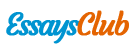# The De Broglie Wavelength

Autor:   •  September 17, 2017  •  648 Words (3 Pages)  •  428 Views

Page 1 of 3

...

The differences for the jump:

deltaE32=3.01*10^-17J=>lamda=6.6nm

deltaE21=1.81*10^-17J=>lamda=11nm

ANSWER: (a) 37 .7 eV; (b) 301 eV; (c) 4.12 nm; (d) 4.12 nm; 6.60 nm; 11,0 nm

6. A ground-state electron is trapped in the one-dimensional infinite potential well with width L = 100 pm.

(a) What is the probability that the electron can be detected in the left one-third of the well (x1 = 0 to

x2 = L/3)?

(b) What is the probability that the electron can be detected in the middle one-third of the well (between

x1 = L/3 and x2 = 2L/3)?

7. The ground-state energy of an electron trapped in a one dimensional infinite potential well is 2.6 eV. What will this quantity be if the width of the potential well is doubled?

En=h^2/(8ma^2)*n^2, where En=2.6*1.6*10^-19=4.16*10^-19 eV ; h=6.625*10^-34 J/s ; m=9.1*10^-31

=>a=1.48*10^7 m

When the width of the potential well is doubled => a=3*10^-7 m

- En= 0.65 eV

8. An electron is trapped in a one-dimensional infinite potential well. For what

(a) higher quantum number and

(b) lower quantum number

is the corresponding energy difference equal to the energy of the n = 5 level?

(c) Show that no pair of adjacent levels has an energy difference equal to the energy of the n = 6 level.

Let the quantum numbers of pair is n and n+1

- En+1-En=((n+1)^2-n^2)*h^2/(8ma^2)=(2n+1)*E1

En+1-En=E5=5^2E1=25E1

=>2n+1=25 and n=12

Therefore higher quantum number is n+1=13

- And lower quantum number is 12

- Now En+1-En=E6

- 2n+1=36=>n=17.5. this is not an integer, so it’s impossible to find the pair for the requirement.

9. An electron is trapped in a one-dimensional infinite potential well that is 100 pm wide; the electron is in its ground state. What is the probability that you can detect the electron in an interval of width Δx =5.0 pm centered at x =

(a) 25 pm,

(b) 50 pm, and

(c) 90 pm?

(Hint: The interval Δx is so narrow that you can take the probability density to be constant within it.)

ANSWER: (a) 0.050; (b) 0.10; (c) 0.0095

...